• CFD, Fluid Flow, FEA, Heat/Mass Transfer

Aero-Acoustics, noise estimation and noise reduction strategies

### CAA: Computational Aero-Acoustics

CAA can be explanded into Computer Aided Acoustics (similar to CAD and CAE) or Computational Aero-Acoustics - both mean same. We may regard sound either a sensation or the stimulus which produces the sensation. The physics is mainly concerned with the stimulus which is the phenomena occuring external to the ear. Acoustics is defined as the science of sound and phenomenon of hearing. While the word 'sound' refers to vibrations of all frequencies (audible or otherwise, similar to the word 'light' which represents visible radiations as well as invisible infrared and ultra-violet radiations), acoustics is reserved for the aspects connected with hearing. (Reference: A Textbook of Sound by A. B. Wood). A book available free is Acoustics: Essential concepts, theory and models of linear acoustics for engineers by Jean-Louis Migeot et al.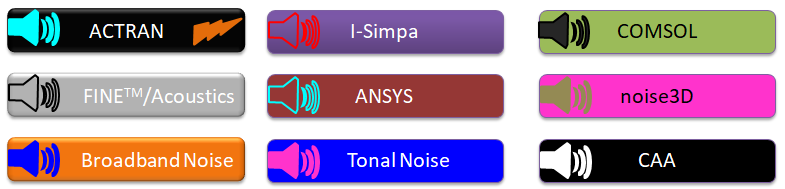Aero-acoustics deals with production, propagation (transmission: air-borne or solid-borne), reflection (as sound moves from one medium to another), absorption, scattering, attenuation and reception (by human ears) of sound waves. The sound is primarily generated by fluid fluctuations forced due to unsteady motion of fluids. Sound is a small perturbation of pressure over a mean (steady state) pressure, p’/p0 which propagates as a wave. Aero-acoustics is different from the study on generation of sound such as organ pipe and loud-speakers. The later is referred as "classical acoustics".

Excerpts from COMSOL user manual: "Acoustics is the physics of sound. Sound is the sensation, as detected by the ear, of very small rapid changes in the acoustic pressure p above and below a static value. This static value is the atmospheric pressure (about 100,000 Pa). The acoustic pressure variations are typically described as pressure waves propagating in space and time." During their propagation, sound waves can be reflected, refracted or attenuated by the medium and can have interference with sounds of different frequencies.

#### Concepts in Acoustics

A detailed mathematical approach to acoustics can be found in this
e-book available free for personal and educational purposes.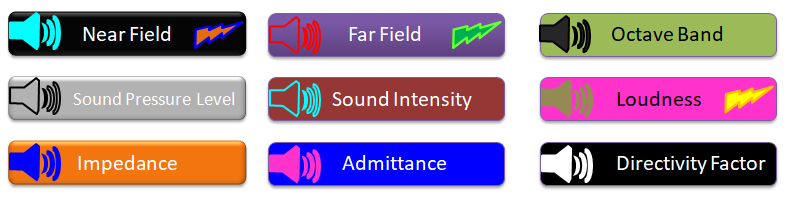• Threshold of Hearing: This it the lowest level of sound intensity that can be sensed (heard) by most humans at a specified frequency of 1000 [Hz]. In air it is 20 [μPa]. The threshold in terms of frequency is the range of 20 - 20,000 [Hz] (threshold of hearing: 0.02 m-Pa, loud noise: 20 Pa in rock-bands, pain is experienced as sound pressure ~ 60 Pa). The peak of the human hearing range is between 1000 ~ 3000 [Hz] (reference STAR-CCM+ tutorial document). Analogous to light wave, sound waves with frequency < 20 [Hz] are called infra-sonic (similar to infra-red) and that of frequency > 20,000 [Hz] are called ultra-sonic (similar to ultra-violet).
• Description of waves: frequency - ν is number of vibrations per seconds, wavelength - λ is the distance after which wave pattern repeats itself, speed of sound c - the velocity of propagation of acoustic waves = ν.λ, wave number - k is the number of waves required to cover a specified distance = 2π/λ. Acoustic waves are characterized by a much lower amplitudes and higher frequency than the pressure waves. Frequency is also known as 'pitch'. For example, a man's voice is low-pitched (fundamental frequency in the band 65 Hz ~ 250 Hz with mean around 120 Hz) and a female's voice is termed as high-pitched (fundamental frequency band 100 ~ 500 Hz with mean around 210 Hz).

Excerpts from "ACOUSTICAL USES FOR PERFORATED METALS: Principles and Applications" - If we play a single key (or three or four adjacent keys near middle-C, the sound energy will be concentrated around the frequency 250Hz (cycles per second).

Approximate sound speeds for 3 media: air, water and steel are 340 [m/s], 1500 [m/s] and 5000 [m/s] respectively. Note that the speed of sound (which is speed of pressure waves) corresponds to fluid in unconfined condition. The speed of pressure waves in a confined space such as long tube is < that in unconfined space due to elasticity of the walls of the pipe. This phenomena is of practical significance in water hammer calculations.

A linear, time-harmonic wave equation in 3D is given by ∇2p' + k2p' = 0 where p' is the acoustic pressure. This is also known as Helmholtz equation for sound pressure.

• The sound pressure p' and the particle velocity u' are the acoustic state variables.
• Particle displacement of a wave traveling in x-direction: y = y0 sin(kx - ωt)
• Particle velocity: u' = dy/dt = -ωy0 cos(kx - ωt)
• Acoustic pressure: p' = -B dy/dx where B = bulk or volumetric modulus of elasticity. Thus, p' = -B.y0k cos(kx - ωt)
• In most of the textbooks, for the sake of brevity wave equations or wave functions are expressed as complex numbers. For example, the general form of an one-dimensional wave function is ψ = A cos(ωt - kx - φ) ≡ A cos(kx - ωt + φ). The complex wave function is ψ = ψ0 e-i[kx - ωt] with complex constant ψ0 = e.
• All these representations complex wave function are based on fundamental mathematical identity, known as Euler's theorem expressed as e ≡ cos(φ) + i sin(φ). In other words: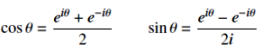• The main advantage of the complex representation over direct real representation is single complex amplitude for both real amplitude and phase angle. This is just a way to present 'real' phenomena using what is called 'imaginary' numbers.
• The equation of sound wave in one-dimension with flow velocity U and Mach number M = U/c is given below. Assuming a time-harmonic solution for the acoustic pressure in exponential form is also given where p'reflected = acoustic pressure of reflected acoustic wave at inlet.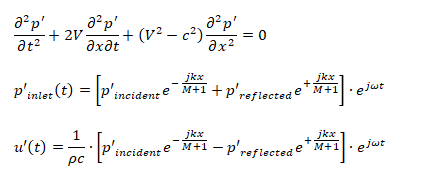• Air Particle Velocity - Acoustics waves are called longitudinal waves which refers to the phenomena that the particles of medium oscillates along the direction of the motion of the wave. Thus, air particles will vibrate about its mean position along the direction of wave propagation. It is usually expressed as RMS (Root Mean Square) velocity [m/s]. Other approach to express it is "volume velocity" for flow inside ducts which equals the product of the rms velocity of the air particles and the cross-sectional area of the tube and has units of [m3/s].

For a given pressure field p'(x, t), the particle velocity is given by u'(x, t) = -p'(x, t)/[ρ·c]

• Noise vs. music: Noise - unwanted sound. Music - pleasant sound. Musical sounds are characterized by smoothness, periodicity and pleasing effect on auditory nerves though not all musical sounds are free from noise. Thus, human perception is important aspect of acoustic studies. Rattle is a type of noise attributed to gears. Squeak is a type of noise generated during heavy braking. Buzz is used to describe a particular type of noise. Whistling is noise generated due to cavities such as sun-roof, Screech is sound generated by shcok waves. Cavitation phenomena in pumps and valves is often detected by crackling noise and this sound is considered the most evident characteristics of the phenomenon. This noise is generated by collapse of vapour bubbles. A musical scale is a sequence of frequencies with a pleasing effects on human ears. Most commonly used musical scale (known as diatonic scale) has 8 frequencies inside an octave with lower and upper band frequencies at 256 [Hz] and 512 [Hz].• Echo vs. Reverberation: The reflection of sound can be measured in time elapsed between the end of the original sound source and time it is reflected back into a room. The duration between these two sound signals defines echo vs. reverberation. If the time gap is > 0.1 [s], the reflection is called echo. If this gap is ≤ 0.1 [s], the reflection is called reverberation. Human ears cannot distinguish between sound signals of less than 0.1 [s]. Therefore, we hear echo as two distinct sound signals and reverberation as one prolonged sound.
• Fundamental Mode - This is the lowest frequency of any vibrating membrane. When a drum (timpani or tabla) is struck, all of the possible frequencies are produced to varying extents but the higher order harmonics (sound waves of higher frequencies), usually decay rapidly leaving only the fundamental. A drum can oscillate at many different frequencies, corresponding to the different eigenvalues, but the fundamental (the lowest) frequency is of the greatest interest since this is the one a listener will hear. An integral multiples of fundamental mode are called 'harmonics' or 'overtones'. Thus, second harmonic is first overtone, third harmonic is second overtone and so on.
• Normal Mode - When a component such as string, membrane or structural parts vibrate at frequency which is an integral multiple of its natural frequency, it is said to vibrate in normal mode.
• Quality - Sounds of the same pitch (frequency) and loudness (intensity) but produced by different devices (such as flute and violin) are distinguished by their 'quality'. Excerts from "A Textbook of Sound" by A. B. Wood: Very few sounds (such as those produced by tuning forks) can be designated as 'pure' or free from overtones, though most of the sounds have a 'predominant' frequency. The presence of these overtones decides the quality of sound. These overtones reveal to the ear the nature of the instrument producing the sound. An analysis of the wave-form of a particular sound reveals which harmonics are present and their relative intensities. Wave-form is therefore an indication of 'quality' of the sound.
• Near Field - This is one of the ways to characterize the qualities of the sound field created by a source in its vicinity. The near field refers to zones closer to the source (typically involving spherical waves and the consequent acoustical behavior). Cabin noise level prediction due to HVAC louvres and flow over outside rear view mirrors (ORVM) are examples of near filed where receiver is closer to the source. The equation of a spherical waves is given by

p' = S·k·c·ρ·/4πr·sin[k(ct - r)], u' = -S·k/4πr [1/k/r·cos[k(ct - r) - sin[k(ct - r)], S = maximum rate of fluid emission from source, ρ is the density of the medium, r is radial distance from source. For spherical waves, the intensity decreases with the square of the distance but the sound pressure level decreases linearly with distance.

• Far Field - This is one of the way to characterize qualities of a sound field created by a source far away from it. The far field involves planar waves and the consequent acoustical behavior. Noise several hundreds of chord length away from a wing of aircraft is an example of far-field situation where flow field may not be required to be modeled all the way till receiver. The dividing line between the two conditions is wavelength (frequency) dependent, in other words the location which signifies transition from near-field to far-field will be dependent of frequency of noise generated. The equation for plane waves is given by

p' = a·k·c2·ρ·sin[k(ct - x)], u' = a·k·c·sin[k(ct - x)], a = particle displacement, k = wave number.

Low frequency sounds have long wavelengths, 340 [m/s] / 50 [Hz] = 6.8 [m] at 50 Hz while the wavelength at 10 kHz is only 34 [mm]. Thus, high frequency waves can be considered planar only after a small fraction of a meter from the source while low frequency sounds require few meters of separation from the source to be safely considered planar.

Real sounds or noise generated from a source may contain many component frequencies leading to a mixture of planar and spherical sources. This makes the measurements and calculation of acoustic field complicated. In theory, plane wave pressures do not decrease with distance as they do for spherical waves.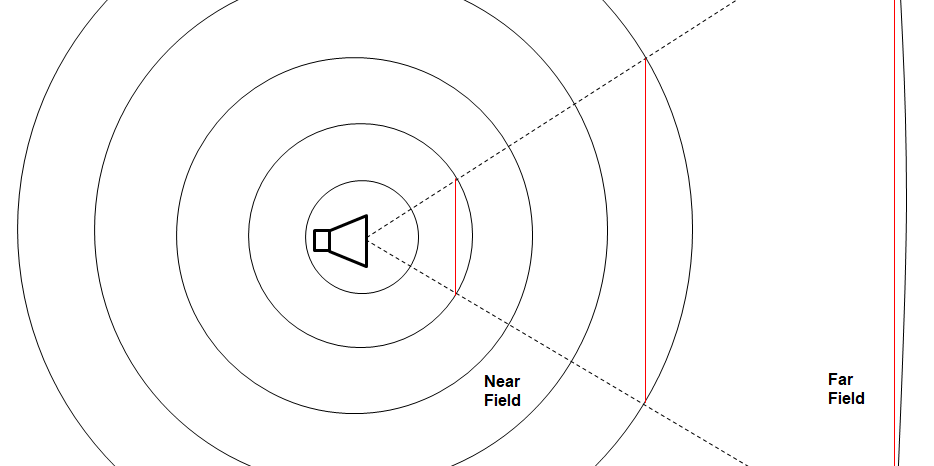• Free Field and Diffuse Field -The terms free field (also called direct field) and diffuse field (also known as reverberant field) refers to sounds measured in a closed zone such as a room. Near the source, the direct sound is dominant while further away the reverberant sound (sound reflected by the walls) prevails.

In direct field (like near field situation), sound pressure decreases linearly with distance while there is no decrease in sound pressure with distance in the diffuse field (as observed in plane waves). The distance at which the direct and reverberant sounds are at equal pressure is called the critical distance.

• SPL - Sound Pressure Level: This refers to the intensity of sound waves. This is represented as logarithm (because sound pressures have large ranges) of a ratio of magnitude of pressure fluctuations to the reference pressure at threshold of hearing and denoted as deciBel [dB]. SPL = 20 × log10(p / pREF) [dB] where pREF = 20 [μPa]. Thus, "threshold of hearing" corresponds of SPL = 0 [dB]. In other form: SPL [dB] = 20 × log10[pRMS] + 94 [dB] where pRMS is in [Pa]. Bel is another measure of SPL and is equal to 10 deciBels (dB). It is dimensionless since it is a ratio of two quantities. Threshold of pain is ~ 130 dB (such as gun-shots, jet engines) and higher levels of the order of 200 dB may occur in explosions, sonic booms, rocket lift-off and similar extraordinarily loud events. Whisper has SPL of 30 dB. Disco and rock concerts has noise level in the range 100 ~ 110 dB.
• For a point source, the sound pressure ∝ 1/r from the measuring point to the sound source. Thus, doubling of the distance, sound pressure will decrease by half.
• log10(2) = 0.301, thus doubling the distance, SPL will drop by 20 × 0.3 = 6 [dB]
• Due to the fact that SPL is based on a log scale, they cannot be added like a simple algebraic summation. As expressed below, adding two sound pressure levels of equal value will always result in an increase of 3 [dB].• Thus, the maximum increase by combination two random noise sources is always < 3 [dB] above the noisier source.
• When the difference in noise level of two source is ≥ 10 [dB], the contribution of weaker noise source is negligible over stronger noise source.
• Example: if a plant have 5 exhaust fans each radiating sound pressure level of 60 [dB]. The sound pressure levels expected with various number of running fans (assuming the noise to be uncorrelated) are:
• 2 fans: 60.0 [dB] + 60.0 [dB] = 63.0 [dB]
• 3 fans: 63.0 [dB] + 60.0 [dB] = 64.8 [dB]
• 4 fans: 63.0 [dB] + 63.0 [dB] = 66.0 [dB]
• 5 fans: 66.0 [dB] + 60.0 [dB] = 67.0 [dB]
• If SPL1 < SPL2 and SPL1 - SPL2 = δ following table can be used to find addition of decibel levels, SPL3 = SPL2 + Δ:  δ 0 1 2 3 4 5 6 7 8 9 10 Δ 3 2.5 2.1 1.8 1.5 1.2 1 0.8 0.6 0.5 0.4
• Sound Intensity - I: Also known as sound-energy flux density, it is the time-averaged value of "[instantaneous sound pressure × particle velocity]" at a point in a temporally stationary sound field (sound levels not varying with time). The particle velocity is the speed at which air molecules vibrate back and forth while transmitting the sound waves. Particle velocity is a vector quantity, while sound pressure is a scalar. Thus, sound intensity is a vector quantity having unit of [W/m2]. Sound pressure level can be measured with a single microphone while sound intensity measurement requires two or more microphones in a specific arrangement.

In a free-field that is where no reflected sound waves are present and far away from a noise source, I = p2RMS/ρc where pRMS is Root Mean Square acoustic pressure.

A similar concept is sound source strength which is the maximum rate of volume displacement [m3/s] produced by a source when emitting a harmonic sound wave.

• Normal sound intensity level - LIN: It equals 10 times the logarithm (base 10) of the ratio of the unsigned value of the normal component of the sound intensity (which is radiated by the sound source under test and determined in a direction perpendicular to the measurement surface) to the reference sound intensity. Like other logarithmic definitions, it is expressed in decibels and the reference sound intensity is 10–12 [W/m2] or 1 [pW/m2].
• LIN [dB] = 10 × log10[I] + 120 [dB] where I is in [W/m2].
• The sound intensity decreases inversely proportional to the squared distance, that is I ∝ 1/r2 from the measuring point to the sound source. Thus, doubling of the distance deceases the sound intensity by 4-times of its initial value.
• Sound pressure (sound field) is not same as sound intensity (sound energy quantity). I ∝ p2.
• Analogy between heat and sound - the sound pressure level, sound power level and sound intensity are analogous to temperature, wattage and heat flux respectively around an electric bulb. Simiarly, important analogy exists between electrical (alternative currents) and mechanical vibration systems. The concept of electrical potential, current and impedance are applied equally well to mechanical systems to explain the production and reception of mechanical vibrations. The pairs inertia - inductance, stiffness - capacitance and friction - resistance represent analogous terms which can be used to explain mathematical theory across the two phenomena.
• Band and Bandwidth - Band refers to a continuous range of frequencies between two limiting frequencies. Bandwidth is defined as range of frequencies, usually of standard size in acoustics. For example octave or one-third octave bands where octave bands are groups of frequencies named by the center frequency having upper limit always twice the lower limit of the range, fc = [fL.fU]0.5. The lower and upper frequencies are also known as the -3 dB or half-power points. Based on the perception of sound by a human ear, the reference middle frequency in acoustics is 1000 [Hz] and octave bands are defined with respect to this value such as (15.6, 31.3, 62.5, 125, 250, 500, 1000, 2000, 4000, 8000, 16000) Hz.
• Centre or middle frequency value f0 of octave band is obtained by successive division and multiplication of 1000 [Hz] by 2. The upper band = f0 × 21/2 and the lower band = f0 / 21/2
• A n-order octave band (such as 1/3 octave) is obtained by successive division and multiplication of 1000 [Hz] by 21/n (e.g. 21/3 for 1/3 octave). The upper band = f0 × 21/2n and the lower band = f0 / 21/2n.
• Loudness: Phons: Loudness is a psychological measure of physical strength (amplitude) of sound dependent collectively on human ear's perception along with sound pressure, frequency, bandwidth and duration. Phon is sound intensity level as a reference frequency of 1000 [Hz]. Two sounds or noise with equal intensity but at different frequencies will not have same loudness. A sound/noise of 75 dB at 1 kHz = 75 phons.
• Designated as Lw, Sound Power Level is the total acoustic energy output of a noise source independent of medium of propagation of the sound (the environment). Lw = 10 × log10(P/P0) where P0 = 1 [pW] = 10-12 [W]. While sound power level is a constant value for each noise source, SPL are dependent on factors such as the distance from the noise source, presence of any reflective surfaces present near the source. Thus, sound pressure levels will always be higher than sound power levels. Sometimes a frequency weighting as per IEC 61672–1 and/or specific frequency bands are applied, this is denoted the A-weighted sound power level - LWA and its unit is denoted by dBA or dB(A).

LW [dB] = 10 × log10W + 120 [dB] where W is in [Watts]. Comparing noise source as a charge, as per Gauss's law: the total electric flux through a closed surface is zero if no charge is enclosed by the surface. Similarly, the total acoustic power through a closed surface is zero if no noise source is enclosed by the surface. Thus, sound power level measurement will not be affected by background noise if the measurement surface encompassing the noise source does not enclose source of background noise.

###### Doppler Effect
It is the change in frequency and wavelength of waves due to
• source or emitter moving with respect to the medium
• observer or receiver moving with respect to the medium
• or even a moving medium.
If the source and observer are moving towards each other the perceived frequency is higher than the actual frequency. Likewise, if the source and observer are moving away from each other the perceived frequency is lower than the actual frequency.Here, 'v' is the speed of wave (sound), 'w' is the speed of the medium and is positive if the wind blows in the direction of sound (source) and negative if it blows opposite to the direction of sound (source). Subscript 'o' refers to the observer and 's' refers to source.
A-Weighting and Equal Loudness Curve: SPL measurements in terms of dB take no account of how sound pressure / power is distributed with respect to sound frequency as it represents a comparison or ratio between the quantity being measured (such as sound pressure or power) and a reference value (reference pressure or reference power). As the sound pressure level (SPL) reduces, human ear's ability o detect low or high frequency noise also diminishes. On the other hand, almost all noises are generally dependent of frequency and consist of a mix of frequencies. A complete representation is a frequency spectrum (dB vs. f curve) where noise levels are plotted as a function of noise frequencies. For purposes like comparing two noise sources, specifying noise reduction level ... a single value is typically required. A-weighted sound pressure levels in terms of dB(A) are widely used for this purpose.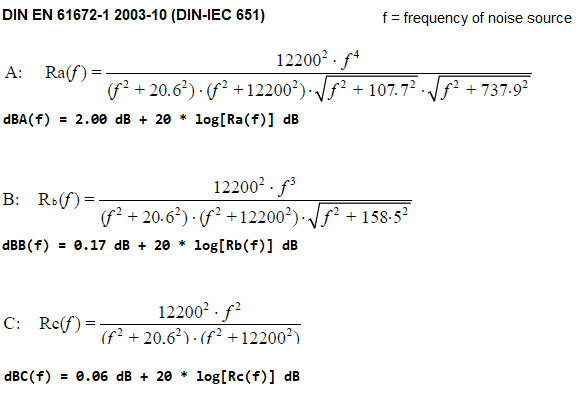The offset values mentioned in formula below (-2.0, -0.17 and -0.06) are to ensure normalization to 0 [dB] at 1000 [Hz] and calculated respectively as 20·log10[Ra(1000 Hz)], 20·log10[Rb(1000 Hz)] and 20·log10[Rc(1000 Hz)].The A-weighting curve describes decibels of 'attenuation' or 'gain' at every frequency over which sound pressure level is measured. Few key features of A-weighting curve are:
• At 1 [kHz], the gain or attenuation is '0' and this frequency value is reference for all other cases.
• Below 1 [kHz], the sound levels are attenuated (diminished) with respect to input levels.
• There is attenuation of 20 [dB] at frequencies 100 [Hz] and 40 [kHz].
• In the range of 1 ~ 6 [kHz], there is a gain of few decibels. The maximum gain is 1.27 [dB] at 2500 [Hz].
• For frequencies > 6 kHz the sound levels are attenuated.
• As gain (that too small relative to attenuation) is limited to a narrow range of frequency, dB(A) value will tend to be < [dB] value in most of the cases but this is not universally true.

Weighting comes from Fletcher-Munson Curves where 'A-weighting' is 40 Phon equal loudness contour 'B-weighting' is 70 Phon equal loudness contour and 'C-weighting' is 100 Phon equal loudness contour.

Steps to calculate A-weighting:

• Adjust the given sound level by the A-weighted adjustments as per graph or formula described above. Note that the subtraction or addition of two decibel values for this particular purposes need to be algebraic.
• Add the sound levels as per the actual addition formula to calculate the overall sound level.• Example calculation: noise levels below 63 [Hz] and above 8 [kHz] are ignored.  f [Hz] 63 125 250 500 1000 2000 4000 8000 Lp: measured [dB] 69 71 73 77 79 78 75 72 Lp: total 84.48 [dB] A-weighting value: δA -26.2 -16.1 -8.6 -3.2 0.0 1.2 1.0 -1.1 Lp' [dB] = Lp + δA 42.8 54.9 64.4 73.8 79.0 79.2 76.0 70.9 Lp(A): A-weighted 83.83 [dB]

Noise Type, Acoustic Impedance and Directivity
• Broadband Noise - This is also called wideband noise - a type of noise whose energy is distributed over a wide range of frequencies of pressure fluctuations (or audible range). Broadband analysis generally refers to noise spectra in the range of 20 - 20,000 [Hz] and narrowband analysis is used to obtain noise spectra in the range 0 - 5000 [Hz].
• Tonal Noise - Tone refers to a specified frequency. Tonal noise refers to sound wave forms that occur at a particular frequency such as RPM of a rotating wheel - also known as narrowband noise. Tonal noise are discrete and occur only at certain frequencies that is the frequency of a tonal noise remains constant for a range of mass flow rates or input flow velocity scale. Sometimes it is also called humming or whining or ticking noise.The image shown above is taken from "Dell Enterprise Acoustics: A Dell Technical White Paper". In the case of tonal stimulation at varied stimulus frequency (f), the ratio of the sound pressure p(f) to the volume velocity Q(f) defines the acoustic behaviour of a system. In a linear system, a sinusoidal excitation produces sinusoidal response which may lead, lag or be in the phase of the excitation. Similarly, the responses produced by a tonal stimulus can differ from the input in terms of the magnitude which can be quantify by a root-mean-square (RMS) value, and phase (which describes the relative timing of the sinusoidal responses - leading or lagging).

• Blade passing frequency: BPF is one of the method to estimated expected tonal frequency component for rotating machines like fas, turbines, blowers, turbochargers, camshaft drive for IV/EV... It is calculated as N/60×nB where nB is the number of blades and N is RPM. This is frequency at which a longitudinal plane is cut through the rotating blades.
• Combustion noise: This is applicable to internal combustion engines where the combustion is not a continuous process and valves open/close at frequency related to operating RPM of the engine (crankshaft rotation). ν = engine crankshaft rotation speed in Hz = N/60 where N = RPM, combustion frequency for a 4 stroke engine = ν/2.
• Tappet noise: A tappet or cam-follower is the part that is made to move vertically by the action of the rotating cam which subsequently causes valves to open. The tappet noise refers to a characteristic hammering or "tak-tak" noise due to clearance between inlet/exhaust valves and corresponding driving or rocker arms.
Ref: Handbook of Acoustical Measurements and Noise Control by C. Harris, 1991 - Fan noise performance can be predicted over a wide range of sizes and speeds using basic scaling relations - Sound Power Level (SPL) = Lw2 = Lw1 + 70 x log10(d2/d1) + 50 x log10(n2/n1) where d is tip diameter of the fans and n represents rotation speed
• Acoustic Impedance - Analogous to the flow of electric current, acoustic impedance of a medium and/or material is the resistance of the passage of sound waves. Impedance is a type of resistance (as we learnt for capacitors and inductors whose resistance is function of frequency of electric current following through them and complicated by the fact that current and voltage may not be in phase). Similarly, acoustic impedance is defined as ratio of acoustic pressure p to acoustic volume flow rate Q. In particular the impedance magnitude |Z(f)| is defined by the ratio of the magnitudes of the sound pressure |p(f)| and the volume velocity |Q(f)|.
• Z = p / Q [Pa.s/m3] ≡ [kg/m4/s] or acoustic Ω. Similar to electric impedance, the flow and pressure may not be in phase and hence like electrical systems, complex numbers method needs to be used to handle such impedances where the real part represents the in-phase component and the imaginary part the out-of-phase component. Imaginary part of the acoustic impedance is called acoustic reactance and the real part of the acoustic impedance is called acoustic resistance.
• The acoustic impedance describes the sound pressure [Pa] needed to produce a unit measure of volume velocity [m3/s] in the tube. For an infinitely long pipe of cross sectional area A which is filled with a medium of density ρ and at temperature T at which speed of sound c = (γ×R×T)0.5, the acoustic impedance is [ρ×c/A].
• The acoustic impedance of a material determines fraction of sound power that will be transmitted and reflected when the wave encounters an interface created by two different materials. The larger the difference in acoustic impedance between two materials, the smaller the amount of transmitted energy will be. Similarly, the acoustic impedance of a sound attenuation device determines fraction of sound power that will be transmitted and reflected when the wave passes through it.
• Characteristic acoustic impedance [Pa.s/m] is defined as product of density of the medium and speed of sound in it. Some authors describe characteristic impedance as ratio of the speed of sound and cross-section area of the duct: Zc = c/A.
• Specific acoustic impedance [Pa.s/m] in a sound field is the ratio of sound pressure and particle velocity [p' / u'] which equals [ρ·c]. This is also known as radiation resistance of the medium and is analogous to Ohm's law where electrical resistance R = ΔV/I, I = current and ΔV = voltage difference.
• Analogous to capacitative and inductive resistance in circuits carrying alternating current, acoustic inertance (where pressure leads the velocity) and acoustic compliance (where pressure lags the velocity) are defined.
• Radiation Impedance - In case of spherical waves, pressure fluctuation p' and particle velocity u' are not in phase which indicates that the acoustic impedance of the medium will have reactance and resistance analogous to alternating currents. The complex notation is used to describe the phase difference in the two types of impedances. Various authors (such as Kinsler et al. 1982, Munjal 1987) have used the expression Zr = R + jX for radiation impedance. The real component of Z is the acoustic resistance. The imaginary component is associated with reactance X.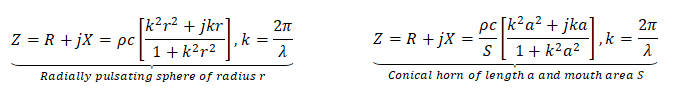Radiation into a free space such end of a duct is expressed as radiation impedance. Here 'R' is the "radiation reactance" which if the energy radiated away from the open end in the form of sound waves and 'X' is "radiation admittance" representing the mass loading of the fluid just outside the open end. As per Munjal, impedance of an unflanged open duct with plane waves propagating inside it is given by: ZUNFLANGED-END = ρc/ADUCT[0.25k2R2 + j 0.6kR].

• Acoustic Admittance - The acoustic impedance and admittance are related quantities that depend on the ratio of sound pressure and sound flow (the volume velocity of sound). As electrical conductance is reciprocal to electrical resistance (impedance), the acoustic admittance is reciprocal to acoustic impedance: Y(f) = 1/Z(f). The units of acoustic admittance are [acoustic siemens], where 1 [acoustic siemens] = 1 [m3/Pa.s]. Admittance and impedance are often collectively refereed as 'immittance'.
• Transmission loss - It is defined as the difference between the sound power incident at the entry of the sound attenuation device such as filter or muffler and the sound power transmitted after the device. Thus: TL = 10 × log(WIN/WOUT) = 20 × log(pIN/pOUT). Transmission loss is measured by mounting a test wall or panel between two reverberation rooms and measuring the sound pressure levels on each side as described below.Note that the pressure fields for TL measurements or simulation case an be generated without a background mean flow - just by using a noise source at one end also known as time harmonic acoustic perturbations.
• Directivity Factor, Q - This is a measure of variation of angular dependence of sound intensity far away from source (that is in a region free of near-field effects). For a spherical waves, the average sound intensity at a distance r is given by IAVG(r) = W/4*πr2. Directivity factor Q is defined as Qθ = Iθ(θ, r) / IAVG(r). Directivity index is defined as DI = 10 × log10[Qθ]. This evaluation parameter is important for speakers where sound source need to cover a large angle without significant drop in SPL.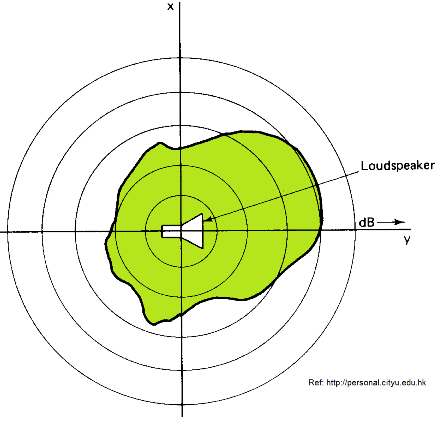• Power Spectral Density - PSD is a representation of the dependence of energy on frequency (or wavenumber). Most often PSD is not a smooth function of time or space and represented in terms of the Fourier Coefficient. Hence, it is also viewed as a distribution of squared Fourier coefficients as a function of wavenumber (or frequency).
• Q-factor: It is defined as Q = f / (f1 - f2). Here 'f' is the frequency of the tone and f1, f2 are the frequencies at the half-power points of the spectral peak. Also, Q-factor = 1/2ζ where ζ is damping ratio.

#### Noise measurements

The measurement of sound levels are performed in what is called anechoic chambers. These are rooms isolated from external sound or electromagnetic radiation sources, use soundproofing on their walls and prevents the reflection of wave phenomena. Anechoic chambers are widely used for measuring the properties of acoustic instruments, measuring of the transfer functions of electro-acoustic devices, testing microphones and performing psychoacoustics experiments. Anechoic chambers are used primarily for SPL measurements, ranking of sound sources, noise source identification and directivity of sound sources and receivers.

ISO 5801:2017 specifies procedures for the determination of the performance of fans of all types except those designed solely for air circulation, e.g. ceiling fans and table fans. Testing of jet fans is described in ISO 13350. The aeroacoustic measurements are performed using a test rig (the so-called In-Duct method) according to the industry norm ISO 5136 where farfield noise levels are recorded inside a circular duct using three slit-tube microphones. ISO 13347 deals with determination of sound power level in industrial fans under standard laboratory conditions.

Benchmarking of simulation results is also one of the important area of measurements in anechoic chamber. However, there can be deviation in test and simulated results due to omission of few noise sources in simulations. For example, in case of an axial flow fan, the deviation may come from additional noise generated from motor fan casing, noise generated by electric motor and vibrations due to unbalanced rotating masses. All these 3 modes of noise source are structure-borne which are generally omitted in CFD simulations intended to estimate fluid-borne noise. A Benchmark Case for Aerodynamics and Aeroacoustics of a Low Pressure Axia Flow Fan - SAE Technical Paper 2016-01-1805 authored by Zenger et al can be used for numerical simulations for noise in fans.

#### Aero-Acoustic Calculations [CFD Simulations]

Excerpts from "Surface integral methods in computational aeroacoustics — from the (CFD) near-field to the (Acoustic) far-field" by Anastasios S. Lyrintzis, School of Aeronautics and Astronautics, Purdue University:

"Aerodynamically generated sound is governed by a nonlinear process. One class of problems is turbulence generated noise (e.g. jet noise). An accurate turbulence model is usually needed in this case. A second class of problems involves impulsive noise due to moving surfaces (e.g. helicopter rotor noise, propeller noise, fan noise etc.). In these cases an Euler/Navier-Stokes model or even a full potential model is adequate, because turbulence is not important. Furthermore, because the acoustic fluctuations are usually quite small (about three orders of magnitude less than the flow fluctuations), the use of nonlinear equations (whether Navier-Stokes or Euler) could result in errors. One usually has no choice but to separate the computation into two domains, one describing the nonlinear generation of sound, the other describing the propagation of sound."

Similarly, excerpts from "Implementation of Acoustical Analogies in Openfoam and CALFEM" by Johan Nilsson: There are several challenges to overcome when attempting to compute aeroacoustical sound. Two of these are the large energy and length scale disparity. The energy in the flow is much greater than the energy in the acoustical waves. To quote Crighton, "If all the acoustic energy radiated in the 45-second take-off of a large jet transport were recovered, that energy would be about enough to fry one egg!". Compare that to the energy in the flow which is used to lift the whole large jet aircraft to get a sense of the energy disparity.

For flow simulations intended to predict noise lelve, mesh needs to be generated keeping in mind upper frequency limit [say 3000 Hz] and at least 10 points per wavelength. Thus, to capture noise up to 5000 [Hz], the maximum element size should be 340 [m/s]/5000[Hz]/10 = 6.8 [mm]. During the transient flow simulation, the time histories of the instant surface pressure values needs to be written into the acoustic data files [*.asd format in ANSYS FLUENT] with which an operation can be performed for the surface zones [such as fan blades] that were selected as acoustic sources.

Excerpts from STAR-CCM+ User Guide: The physics in aeroacoustics are inherently transient and ultimately, must be solved using transient calculations. The computational run times that are associated with such simulations are high. As such transient simulations take a long time to run, optimize the mesh and settings so that you only capture noise sources and frequencies that are relevant to your analysis. An initial steady state analysis provides you with the data for optimizing the mesh and settings.

Recommended Analysis Procedure: It is recommended that aeroacoustics simulations are set up as three-dimensional cases, using compressible flow rather than constant density and run:

• Set up and run the case in steady state mode using a relatively coarse mesh to optimize the case for aeroacoustics. Use the broadband* noise source models to identify where to refine the mesh. Use the mesh frequency cut-off scalar function to judge the required size of the cells in the source region.
• Load the case setup in Step 1. Refine the mesh using information from the broadband noise models and the mesh frequency cut-off scalar function. Run the case again in steady state mode, restarting the solution from that of Step 1.
• Load the case setup in Step 2 and modify it to be a transient simulation with an appropriate time-step [approximately 10 points per wave-length in the source region]. Choose LES-based or DES-based turbulence modeling and restart the solution from that of Step 2. To be able to post-process Lighthill Stress Tensor field functions, select the Lighthill Stress Tensor. For far field propagation analysis, use the Ffowcs-Williams Hawkings (FW-H) model.
• Run the simulation. To predict noise level using FW-H method, acquire samples at approximately 1 to 5 [Hz] spacing in frequency domain.

*In situations involving calculations of broadband noise, statistical turbulence quantities computed from [steady state] RANS equations - mean flow field [u, v, w, p], turbulent kinetic energy [k] and turbulent dissipation rate [ε] - is utilized, in conjunction with semi-empirical correlations and acoustic analogies, to estimate source of broadband noise. However, this model is limited to the broadband noise characteristics prediction and does not provide any tonal performance data.

##### Acoustic sources

James Lighthill in the 1950s using free flow assumptions (no reflections in solid surfaces) developed a wave equation including a source term caused by the instantaneous velocity and pressure fluctuations in the flow. He used the the same set of governing equations which describe flow field for fluids. The way to solve the acoustic wave equation is by integration of the sources using Green functions. This analogy holds true for cases like noise generated by jet engines in aeroplanes but may not be appropriate for closed volume like automotive passenger cabins. Lighthill established aeroacoustics when he developed an equation for the propagation of sound waves for a turbulent flow. Since then many extensions to Lighthill’s original theory have been made. Some of the renowned are Curle’s analogy that takes into account the presence of solid boundaries and Ffowcs-Williams and Hawkings (FWH) equation that also includes surfaces in motion.

Noise is a sort of very low amplitude pressure wave typically measured at a significant distance away from the source. To model this, a very fine mesh is needed all the way to the measuring or receiver location and beyond that to avoid reflection of sound wave. Since, the wave is a transient phenomena, a time-accurate solution of flow field is required.

The limitation of computational resources are very effectively addressed by aeroacoustic analogies by reducing the computational power needed when solving for sound propagation. Acoustic analogies helps trim the computational domain to the near field where high resolution is required and the sources are computed. All non-linear effects are prescribed to the near-field computation and a linear wave operator is used to compute propagation of the sound in the acoustic domain.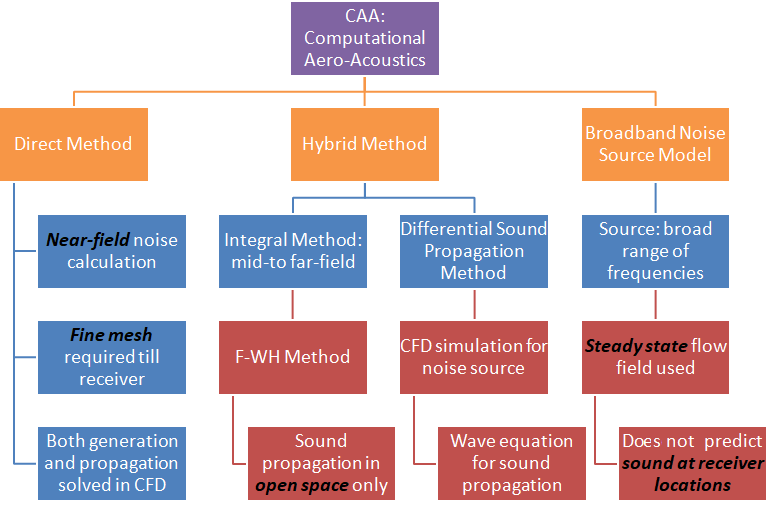Direct method Intgral Method Governing equations capable of modelling viscous and turbulence effects need to be employed Unsteady NS equations such as DNS, RANS and filtered equations used in DES and LES Sound propagation is directly resolved, compressible form of governing equations (e.g. compressible RANS and filtered equations for LES) needs to be solved Computationally difficult and expensive as it requires accurate numeric, very fine meshes all the way to receivers acoustically non-reflecting boundary conditions. Near-field flow obtained from unsteady RANS equations, DES or LES approach To predict the sound, analytically derived integral solutions to wave equations is used FLUENT adopts time domain integral formulation wherein time histories of sound pressure at receiver are directly computed by surface integrals Time-accurate solutions of the flow-field variables (pressure, velocity and density) on (noise) source surfaces are required to evaluate the surface integrals URANS, LES or DES required Both broadband and tonal noise can be predicted FW-H model cannot be used to predict noise propagation inside ducts or wall-enclosed space

Turbulent flow is considered in the source region and used as excitation to the acoustic propagation. The sources are categorised as quadrupoles (within the field such as in turbulent wakes - derived based on time history of velocity field), dipoles (on stationary surfaces with flow-induced fluctuating pressure - derived based on time history of the static pressure on the surfaces), monopoles (mass-flow fluctuation across a boundary such as wall or when mass is added at an unsteady rate: in turbomachines it is called thickness noise due displacement of fluid caused by motion blades having finite thickness) and rotating dipoles (fluctuating pressure on moving surfaces such as blades of a fan and turbines, also known as loading noise - derived based on time history of the static pressure on the surfaces). A monopole source [1/s2] can be thought as point source which has a equal intensity in all directions - a radially oscillating sphere. A dipole source [N/m3] is stronger in two opposite directions and can be visualized as translational oscillating sphere.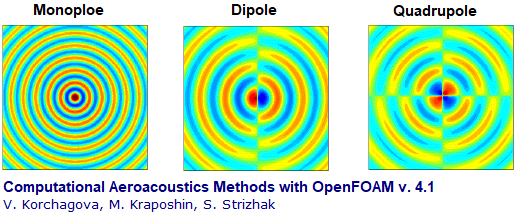Thickness noise: As the rotor rotates, the volume of each blade displaces fluid volume and consequently causes pressure fluctuates in near field thus noise is generated. This noise is tonal at the running frequency and generally weak for fans running at low speed say 1000-2000 [RPM].

Further explaination is given in this page and few images from this reference are shown below: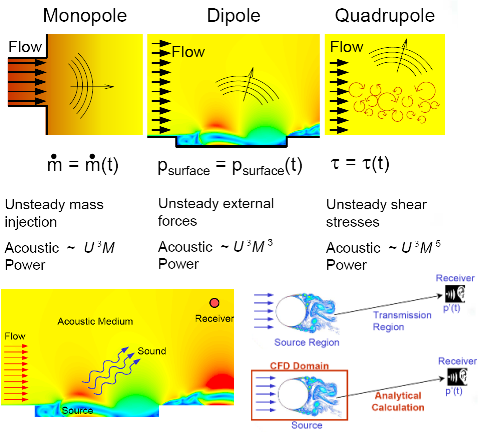Direct Method: Any acoustic calculation approach requires at least 2 steps: generation and propagation of sound. In direct method, both generation and propagation of sound waves are directly computed by solving the fluid dynamics equations modified to capture sound waves. This method requires very fine meshes encompassing receivers as well as acoustically non-reflecting boundary conditions.

Hybrid (CFD/CAA) Approach: This is called so because noise predictions are a two-step approach due to the large difference in requirements on the flow field and acoustic propagation. Ffowcs Williams-Hawkings formulation (bounded flow) and Lighthill's analogy (unbounded field) are examples of hybrid approach. A distinction will be made between calculating the flow field and using the data from the flow field to predict the sound field. Full-fledged CFD tools are used to find the source term and Linearized Euler Equations (LEE) is used to compute the sound propagation.

• Step - 1 : Calculate the source of noise - this is typically achieved by a 3D simulation in a CFD program, either using RANS or LES approach. The Acoustic Analogy approach introduced by James Lighthill is used to divide computational domain into a non–linear source region and a wave propagation region.

CFD simulation can be either of the followings. ANSYS FLUENT recommends LES turbulence model because it resolves all eddies with scales larger than grid scale and hence wide-band aeroacoustic noise can be predicted correctly using LES model. Similarly, to calculate pressure fluctuations on the wall, PRESTO! is recommended for spatial discretization of pressure as it is more accurate scheme for interpolating face pressure values from cell pressures. FLUENT also recommends "Bounded Central Differencing" for momentum in case of LES on unstructured mesh.

• Incompressible, transient
• Compressible, transient
• Step - 2 : Extract the noise sources using Lighthill's analogy for low Mach number flows and Mohring analogy for high Mach number flows. The fluid flow domain uses a finer mesh than the acoustical mesh. The later may include any objects that is required to see the effect of their shape and size in the acoustical domain. Also, the fluid and the acoustical domains do not need to encompass the same space and acoustic domain is usually always bigger than the fluid domain.
• Step - 3 : Use CAA tool such as SYSNOISE, ACTRAN, NUMECA to solve for noise propagation and SPL results. It is not necessary to specify a rigid wall boundary condition in an FEM acoustic analysis, as it is the default boundary condition in acoustics (analogous to default no-slip boundary condition in fluid flow simulations).
A process flow based on user manual and tutorial examples in ANSYS FLUENT can be summarized as per steps described below:Broadband Noise Source Model: In turbulent flows with no rotating components like fans and blowers, sound energy is a continuous distribution over a broad range of frequencies. In other words, no distinct spikes in sound levels are present at few discrete frequencies only. In such cases turbulence quantities [velocity field, turbulent kinetic energy - k and the dissipation rate - ε] computable from RANS equations can be utilized to estimate source of broadband noise. However being a near-field approach, the model does not predict the sound at receivers far away from the flow field. This model can be employed to extract diagnostic information on the noise source to check portion of the flow primarily responsible for the noise generation.

This method available in ANSYS FLUENT quantifies the local contribution (per unit surface area or volume) to the total acoustic power generated by the flow and does not require transient solutions to the flow field.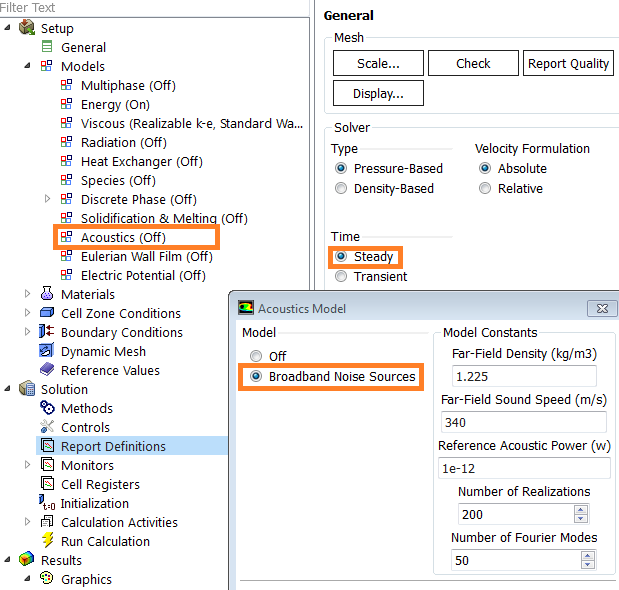The FWH method requires a transient simulation with timesteps lower than highest frequency of sound to be resolved. For time step δt, the highest frequency computed in acoustic analysis is f = 0.5/δt#### Ways of Attenuation of Sound Levels

• Note on source of noise in turbomachines:
• For low-speed rotating machinery, the contribution by quadrupole sources is low and can be neglected. However, loading noise is expected to be main source of noise.
• In case blade is very thin, contribution of noise due monopole sources is insignificant.
• Helmholtz Resonator: Here δEND is end-correction (similar to organ pipes). Acoustic impedance at the inlet (or opening) of an HR, ZHR = p'/ANECK/u'.It is equivalent to a spring-mass-damper system where 'spring' is represented by cavity volume and 'mass' is air inside the neck of the resonator. δEND is an additional length to the actual length of the neck and accounts for the entrained mass of the fluid that vibrates outside the opening of the neck. The multiplication factor 2 refers to two openings though the value of end-correction at the two ends are typically different. LEFF = LNECK + LEND-CORRECTION.

End correction at neck-cavity interface

• As per "Munjal, M.L. 1987, Acoustics of Ducts and Mufflers", at exit of straight ducts: LEND-CORRECTION-CIRCULAR-DUCT = 0.6RNECK.
• As per "Selamat, A. and Ji, Z.L. 2000, Circular asymmetric Helmholtz resonators, Journal of the Acoustical Society of America, 107 (5), 2360–2369", end correction LNECK-CAVITY = 0.82×RNECK [1 - 1.33RNECK/RCAVITY].
• As per Ingard, LNECK-CAVITY = 0.8/3π×RNECK [1 - 1.24RNECK/RCAVITY].

End correction at neck-duct interface: as per "Ji, Z.L. 2005, Acoustic length correction of a closed cylindrical side-branched tube, Journal of Sound and Vibration, 283, 1180-1186"

• for RNECK/RDUCT ≤ 0.40:• for RNECK/RDUCT > 0.40:• As per Onorati, the end-correction value is 0.3RNECK valid for f ≤ 500 [Hz]. As per Rayleigh, the end-correction value is 0.85RNECK valid for 500 ≤ f ≤ 1500.

Ref: D. W. Herrin, Ph.D., P.E. University of Kentucky, Department of Mechanical Engineering: Transmission loss of a HR with anechoic termination is specified as:If the cavity of resonator is replaced with a pipe similar to its neck, a Quarter-Wave Resonator results. It has an effect similar to the Helmholtz Resonator. Both HR and QR are also called "side-branch resonator" which are analogous to tuned dynamic (vibration) absorbers. These resonators attenuate sound in the main duct and TL is confined in a narrow band (band-pass filter) centered at the natural frequency of resonator.

HR frequency and TL calculation (with anechoic termination downstream the HR neck) is described below. The Excel file can be downloaded from here.Excerpts from "A detailed tutorial for evaluating in-duct net acoustic power transmission in a circular duct with an attached cylindrical Helmholtz resonator using transfer matrix method" by Singh et al.: When a HR is mounted on to a duct, a coupled system is created whose resonance frequency is different to that of the HR as a stand-alone device. Therefore, the frequency at which the maximum reduction of in-duct net acoustic power transmission downstream of a HR occurs does not correspond to the resonance frequency of the HR.
• Impedance of an HR: In the following derivation using transfer matrix method (TMM), subscript N refers to the neck and subscript C refers to the cavity. For neck, length is the effective length including end-correction.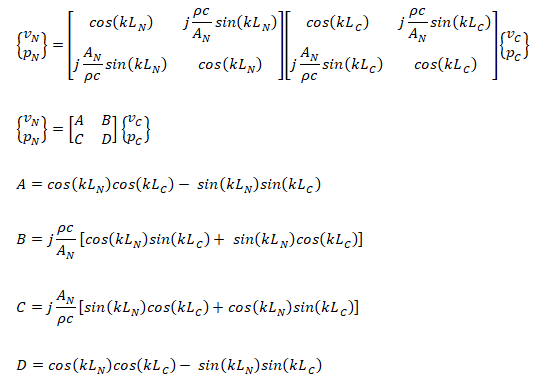At the end of the resonator cavity, due to rigid termination, there will be no flow and hence vC = 0.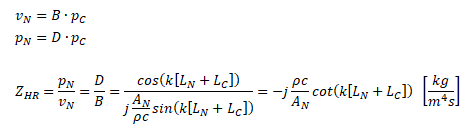The negative -j indicates the fact that the acoustic pressure and acoustic velocity are not in phase.
• S. Tang, “On helmholtz resonators with tapered necks”, Journal of Sound and Vibration, Vol. 279, no. 3, pp. 1085 – 1096, 2005.• Flow expansion chambers: contraction and expansionThe transmission loss of an expansion chamber is given as: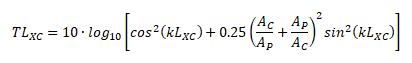Here: LXC and AC are length and cross-section area of the expansion chamber respectively, AP is the cross-section area of inlet pipe. Similar to HR, expansion and contraction end corrections need to be accounted in calculation of effective length of the expansion chamber. This correction length for abrupt area changes are not dependent on frequency of sound waves but a function of geometry only and is valid for both zero flow (only sound wave) and non-zero mean flow. Note:
• Acoustic inlet pipe length = geometric length + end correction at both ends.
• Acoustic length of chamber = geometric chamber length – 2 × end correction.
• Acoustic length of outlet pipe = geometric length + end correction at both ends.
Various variants of expansion chamber are used to improve the TL such inlet extension, outlet extension, flow reversal, extension with a bend ... TL value reported in "Muffler Modeling by Transfer Matrix Method and Experimental Verification" by S. N.Y. Gerges etal. is shown below.• Acoustics Filters: Like a coffee or tea filter which lets only particles below certain diameter, an acoustic filter blocks some components of sound. Soft cushions on our ear phones are sound filters to some extent. These filters are categorised based on the frequency of sound they block or allow to pass. A low-pass acoustic filter will block the high-frequency components of a wave and allow to pass through only the low-frequency components. Similarly, a high-pass acoustic filter will block the low-frequency components of a wave and allow to pass through only the high-frequency components. A pass-band filter has two cut-off frequencies and is a combination of low-pass and high-pass filter.

#### Mufflers (Silencers) and Screens

There are two ways to attenuate the level of sound: reflective systems - here the incident sound is scattered and canceled by destructive interference and dissipative system - here the incident sound energy is absorbed and hence has to be converted into the heat. Expansion-contraction chambers, resonators and Herschel-Quincke tube fall under the category of reflective systems.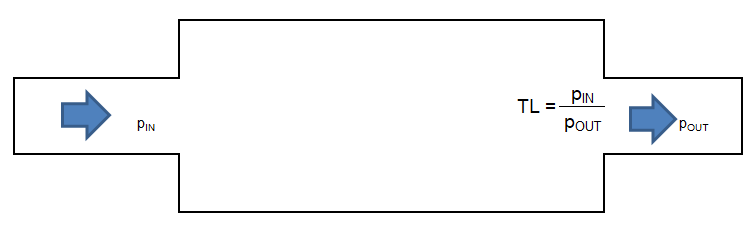Automotive exhaust mufflers use perforated tubes. The STEP file and FreeCAD file of this geometry can be downloaded for mesh generation and simulation.There are two basic types of mufflers or silencers:
• Reflective or reactive mufflers - the operating principle is to reflect acoustic waves by abrupt area expansions or changes of impedance. Such mufflers are recommended for the low frequency range where only plane waves can propagate in the system.
• Dissipative mufflers - these type of mufflers dissipate acoustic energy into heat through viscous losses either in fibrous materials and/or flow-related hydraulic losses such perforated pipes shown above. This type of muffler is recommended for higher frequency ranges.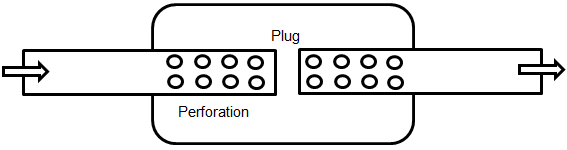The plug flow muffler shown above works at all frequencies by dissipating sound energy when waves formed due vortices at perforation interfere with the waves of the sound field. Thus, the porosity (fraction of void space) and flow velocity through the holes will decide the performance of such mufflers. The perforates become more effective in automotive exhaust systems where flow is pulsating (as opposed to a constant mean flow).
• If the radius of the holes perforation is ≤ 1 [mm], it is a micro-perforated panel. [Reference: Temiz, M. A. (2017). Passive noise control by means of micro-perforated plates : developing tools for an optimal design Eindhoven: Technische Universiteit Eindhoven] Micro-perforated plates (MPPs) are plates with orifices whose diameter is of the order of 1 [mm] and the open area to the plate surface ratio i.e. porosity (σ) is of the order of 1%. The distinction between an MPP and a regular perforated plate is made through the definition of the Shear number 'Sh'.
• Shear number is the ratio of the perforation radius to the oscillating viscous boundary layer (Stokes layer) thickness, Sh = dp / [2δv].
• Stokes layer thickness is δv = (μ/ρ/ω)1/2, where μ is dynamic viscosity of acoustic medium and ω is angular frequency of sound excitation. In an MPP, at lower frequencies the perforations are more than 50% covered by Stokes layer, that is Sh ~ 1.0. Vortices form at the edges of the perforations but they remain local - vortices are not blown away by acoustic velocity.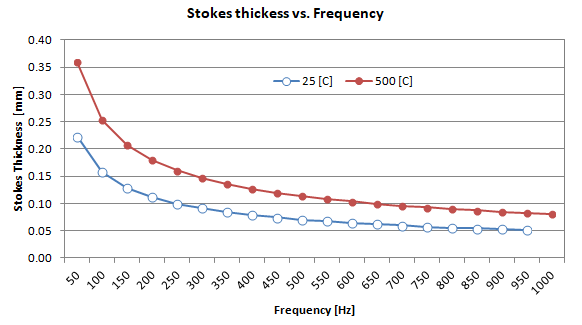• There is no clear boundary, however if radius exceeds 10 [mm] – it is a perforated panel.
• Small diameter holes result in better absorption. The absorption increases with decrease in open space of panel.
• Perforated plates are also designated by Transparency Index (TI) defined as n·d2/t·b2 [reference: ACOUSTICAL USES FOR PERFORATED METALS: Principles and Applications]. Here 'n' is number of perforations per unit area, 'dp' = perforation diameter, 't' = sheet thickness, 'b' = shortest distance between holes = h – d, 'h' = centre distance between two adjacent holes. Different types of perforation are shown in following image:• The the entire cross-section of plate is not uniformly perforated, porosity and perforation rate are different. The porosity 'σ' is defined as the total area of the perforated holes divided by the area of the plate. The perforation ratio is the area of a single hole divided by the polygon area where it is inserted (which gives the influence between hole interaction, no interaction is assumed in φ < 50%).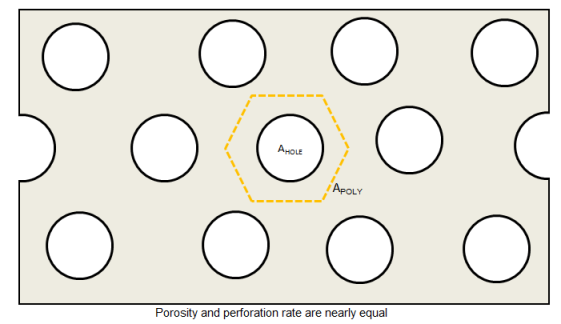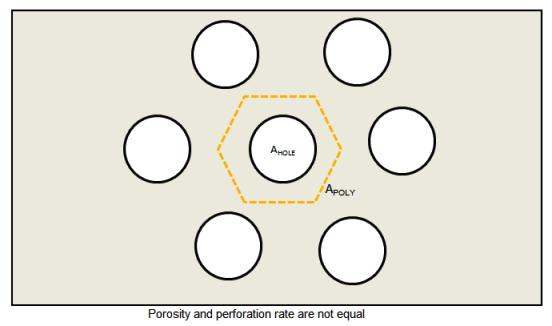• As per D. Y. Maa, "Potential of microperforated panel absorber", the Journal of the Acoustical Society of America, vol. 104, no. 5, pp. 2861–2866, 1998, ZMPP = RMPP + j XMPP.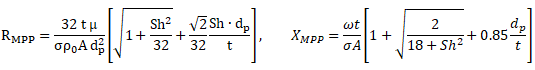• The sound attenuation level for sheets with varying TI is plotted below. It is evident that at frequencies below about 1000 Hz there is little attenuation: the sound passes right through most sheets with no loss whatever.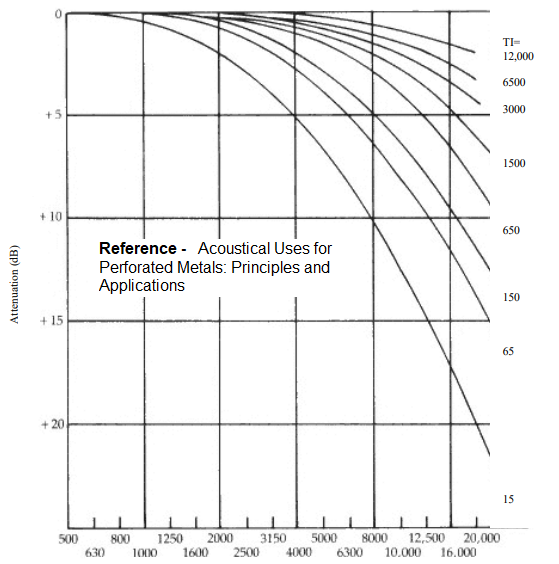• In aero-acoustics calculations of screens (wire-mesh) are modeled as porous media with rigid skeletons. Many models have evolved for such geometry and Johnson–Champoux–Allard (JCA) model is one of them. Two independent parameters are required to estimate the acoustical behavior of a screen: the (hydraulic) radius of the perforations or opening 'r' and the open porosity (or perforation rate) 'σ'. Note that for screens, open-porosity equals the perforation rate defined for MPP above. The factor tortuosity is used to account for flow distortions due to the tortuous path of flow and wave in such porous media. The dependent parameters are (static) flow resistivity = 8μ/φ/r2 where μ is the dynamic viscosity, characteristic viscous length Λ and characteristic thermal length Γ. For material having identical and parallel cylindrical perforations with (nearly) circular cross-section, Λ= r and Γ = r.
• There are various empirical models implemented in CFD simulation tools such as COMSOL to model propagation of acoustic waves in porous materials. These are known as Poroacoustic Models sch as JCA, Biot-Allard model and Williams EDFM (equivalent density fluid model) for visco-inertial dissipative effects inside the porous media. Most poroacoustic models are only valid in the rigid [matrix is assumed to be infinitely stiff] or limp [stiffness so low that it cannot support free, structure-borne wave propagation] porous matrix approximations. Further reading: J. F. Allard and N. Atalla, Propagation of Sound in Porous Media: Modelling Sound Absorbing Materials. 2nd ed. (Willey, New York, 2009), pp.16-106.
• Most of the systems have noise components varying from low to high frequency range and hence no single type of muffler serves the purpose. Hence, dissipative mufflers can be made of hybrid type where flow losses can be used to work also at low frequencies. For example, automotive exhaust mufflers are of hybrid type using a combination of reflective and dissipative muffler elements where reflective part removes low-frequency engine noise generated by combustion and valve motion while the dissipative part is designed to take care of higher-frequency noise.
Transmission and Insertion Loss for a Muffler: As described in the schematic below, the gas pressure in the duct is the result of superposition of waves coming from the source and reflections from the muffler cavity, it is impossible to directly measure the incident components of the pressure pulsation into the muffler. At the same time, all acoustic frequencies of interest (typically in the range 20 ~ 2000 Hz) must be excited which requires a need of large-band acoustic source during the test. Anechoic termination is also known as reflection free termination.

TL is defined as difference in sound power between the incident wave entering and the transmitted wave exiting the muffler when termination is anechoic (no reflecting waves present - i.e. the acoustic pressure of reflective wave at the outlet is 0 - p'outlet = p'transmitted). However, inline with other definition, it is calculated as logarithm of the ratio of sound powers. Attenuation is the difference in incident and transmitted sound powers through the muffler but the termination need not be anechoic. Thus, attenuation is depedent on outlet pipe length. TL is independent of the inlet and outlet pipe length and depends only on the geometry of muffler.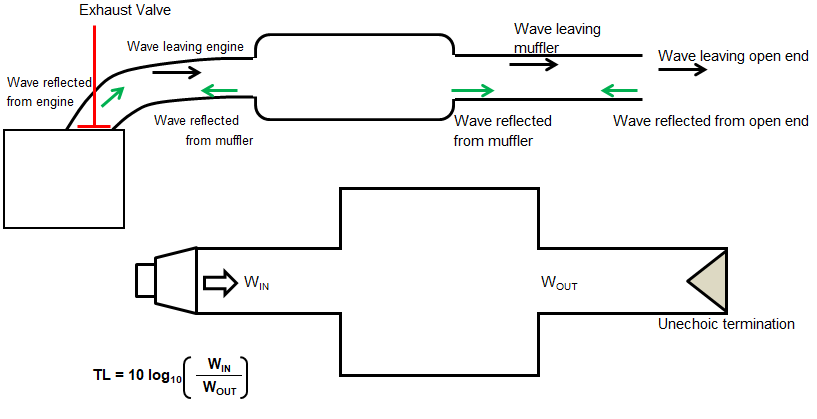Insertion loss = SPL1 - SPL2 which is the difference between the radiated sound power with a reference exhaust system (without any muffler) and the radiated sound power with a muffler system. IL is dependent on both design the muffler as well as the impedances of source and the radiation.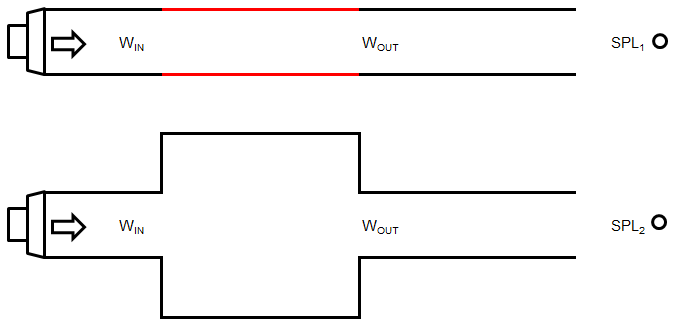Similarly, pressure loss (PL) is an important performance parameter which depends only on the shape and size of the muffler and is defined as difference between the (static) pressure drop across the muffler. The sound (or acoustic) pressure level difference across the muffler is termed as Noise Reduction (NR).

 Design parameters Insertion loss Transmission loss Dependent on geometry? Y Y Influence by source impedance? Y N Dependent on radiation impedance? Y N Anechoic termination required? N Y Calculation Difficult Easy Measurement Easy Difficult
Effect of mean flow: The effects of mean flow on silencer acoustic performance are: first is to affect the sound propagation in the silencer, the other is to induce aerodynamic noise due to flow fluctuations and vortices (turbulence). In silencers, the typical mean flow Mach number is < 0.3 and hence the aerodynamic noise generated due to mean flow turbulence can be assumed negligible. Influence of mean flow on the sound propagation in the silencer can be explained as the convective and dissipative effects. The dissipative effect of medium viscous (especially in the orifices), which can cause sound energy loss and thus increase TL.

#### Noise Control Methods

Analogous to HVAC (where cooling effectiveness is achieved by reducing heat load and maintaining uniform cooling), most of the noise control techniques use a combination of 'absorption', 'reflection' and "transmission or diversion of sound" energy through air-borne and structure-borne paths. Such arrangements and walls are evaluated in terms of "transmission coefficients" and "sound transmission loss" values.
• Transmission coefficient is a frequency-dependent physical property of noise-attenuating materials define as τ = intensity of transmitted sound / intensity of incident sound energy. It also denotes ability to reflect or block sound energy.
• An ideal reflecting wall or material has transmission coefficient = 0 that is STL = ∞, the transmission coefficient of an opening is 1.0 that is STL =0.
• Sound transmission loss is logarithm of inverse of transmission coefficient: STL = 10 × log101/τ
• Sound absorbing materials allow sound waves to enter into its skin and dissipate the energy through viscous dissipation. Such materials are generally porous such as foam and laminates.
Steps to control noise: Determine noise sources using intensity meter or sound camera (an array of microphones) and identify air-borne, liquid-borne (cavitation) or structur-borne (vibration) sources. Note that the measurement of noise radiating from a particular machine can be accurately measured only when the background noise is lower by more than 3 [dB].
• Noise control through air flow
• Reduce pressure drop or flow rates at outlet grilles / louvres
• Avoid barriers or obstacles in the flow
• Improve geometry for smoother flow: elbow bend instead of sharp knee bend, use of screens and separators in bends
• Noise control for structure-borne noise
• Check and correct imbalances in rotating parts
• Use sealing rubbers near mounting of protective covers such as belt or chain drives
• Use flexible hoses to reduce transmission of vibrations
• For solid particle handling devices (sitteres, mixers, blenders): increase impact time (reduce speed) and decrease impact/collision speed, minimize impact mass by using smaller particles and increase mass of housing of a mixing tank.
• Select appropriate bearing keeping in ming vibration levels, proper lubrication also dampens the vibration.
• Use acoustic (perforated) screens - single or multi-layered with a layer of sound energy absorbing (damping) material attached towards sound energy source.
• Stiffen the panels by cross-ribbing

Exceprts from build.com.au/reflection-diffusion-and-absorption-sound: Reflection is often used to redirect noise from outside - consider highway barriers, which reflect traffic noise into the sky. If you can always control the way sound is reflected then this type of soundproofing can be effective. Reflective barriers are a good way to block out exterior noise.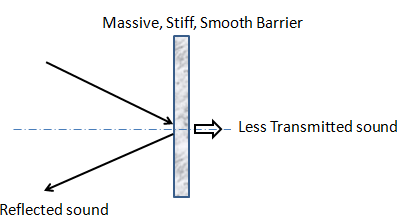Diffusion is a great way to prevent echoes, dispersing the sound wave in all directions when it hits an irregular surface. Think about how much of a difference carpet or a wall rug can make in a brick or concrete room. This method is very effective for high to medium frequencies, as the vibration strength is less than that of a low frequency sound, and therefore easier to disperse.

Absorption performance varies a lot based on the frequency of sound and the absorptive capabilities of the material. A commonly used sound absorber is the underlay in carpet; this works to draw energy from the sound wave and convert it into a tiny amount of heat, creating that ‘deadening’ of a sound. Absorption works best in mid to high frequencies - lower frequency sounds can push through with more force.The PDF file can be downloaded from here. The sound absorption coefficients as reported by Department Of Commerce, Bureau Of Standards, Washington, Letter Circular 293, November 28, 1930 can be downloaded from here.

#### Applications of Aero-Acoustics - CAA

• Automotive Exhaust Silencer (UK English) or Mufflers (US English): intake and exhaust noise can be due to pipe resonance, shell vibration and turbulent fluctuations
• Air intake ducts and air cleaner / filters housing for combustion engines emit acoustic noise mainly based on turbulences and their interaction with solid surfaces
• Cabin Noise from HVAC module: tonal component due to blowers, broadband component due to turbulent flows and shell vibrations
• Instrument panel vibration, ORVM (Outside Rear View Mirror) and A-Pillar noise
• Rotating devices: Compressor and Fan noise, high frequency noise in turbo-chargers - whistling, structure-borne noise
• Vacuum cleaners
• Building air-conditioning Systems: duct noise, sound at exits from grilles (louvres), design of concert halls and conference rooms where an even distribution of sound pressure is desired

#### Numerical Tools for Aero- and Vibro-acoustics

1. LMS SYSNOISE (now part of Siemens): Based on Boundary Element method (BEM) which is a well established numerical method for solving acoustic radiation problems in unbounded domains. It can be coupled with many CFD softwares such as FLUENT.
2. ACTRAN by MSC Software Corporation: For example: process flow chart for a vibro-acoustic simulation in ACTRAN is: Multi-body-dynamics (MBD - ADMAS) → NASTRAN → ACTRAN for structure-borne noise. An example screenshot for aero-acoustic simulation for ORVM noise in cabin of a car is3. ANSYS FLUENT, Mechanical and Multi-physics, Acoustics ACT Extension: FLUENT is used primarily for acoustic field generation using broadband noise and FWH analogy. Mechanical and Multi-physics modules can be used for harmonic response analysis and aero-vibro-acoustics. FLUID29 and FLUID30 are used to model the fluid for 2-D and 3-D cases respectively. FLUID129 and FLUID130 are companion elements to FLUID29/30 for modeling far-field or infinite envelope (absorbs incoming pressure waves) around the FLUID29/30 elements.

Broadband noise source calculated in ANSYS FLUENT for flow over a bluff body is shown below.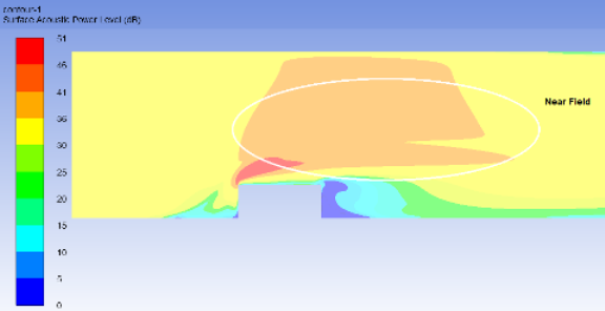ANSYS FLUENT also has "Jet Noise Source Model" based on work of Goldstein to account for anisotropy of turbulence in axisymmetric turbulent jets.
4. OpenFOAM: The official release has post-processing utility called 'noise' for noise analysis of pressure data. The noise post-processing utility requires pressure data in a file which can be generated either by probe function at few select locations or using 'sample' utility. The later can be used to write or export pressure fluctuation data over a patch or surface using sampleDict utility. This is required to calculated noise from a surface source.

OpenFOAM-dev has a library called libAcoustics far-field noise computation which can be accessed from here. incompressible\ pimpleFoam\ LES\ vortexShed contains example of noise calculation. Martin Heinrich from Institute of Mechanics and Fluid Dynamics, Freiberg University of Mining and Technology has implement the modified Curle acoustic analogy presented by Larsson et al. as functionObject for OpenFOAM 3.0.x. The method is implemented for incompressible cases including the surface and volume integrals. The library is available at https://github.com/Kiiree/curleAnalogy The description of this implementation as per slides prepared by Aya Aihara from Division of Electricity, Uppsala University, Sweden can be found here.

5. GT-Power from Gamma Technologies, Wave from Ricardo and BOOST-SID from AVL: Primarily focused on automotive systems for noise calculations in engines, HVAC systems and gear chattering in gear-boxes. BOOST-SID is a linear acoustics frequency domain solver based on transfer matrix method to calculate acoustic properties of a system. A BOOST SID model is made up of a source, a system and a termination. The term 'system' refers everything in between the source and the termination.
6. NUMECA: General purpose CFD software with acoustics capabilities named FINETM/Acoustics. It can calculate tonal noise, broadband noise sources and its propagation with Boundary Element Method (BEM) / Finite Element Method (FEM). It also has Ffowcs Williams-Hawkings (FW-H) solver for radiation analysis with capability to import unsteady flow field data from most commercial CFD tool. It computes vibro-acoustic noise through fluid-structure coupling by importing structural modes from NASTRAN, ABAQUS and ANSYS.
7. COMSOL: It is a general purpose multi-physics solver which includes acoustics capabilities. COMSOL has two interfaces, transient interface and frequency domain interface for modeling the sound waves in the time domain and frequency domain respectively. Transient interface computes the pressure variation by modeling the propagation of acoustic waves in fluids at quiescent background conditions. Frequency Domain interface computes the pressure variation for propagation of acoustic waves in fluids at quiescent background conditions. It is suited for all frequency-domain simulations with harmonic variations of the pressure field.

Both ANSYS Mechanical and COMSOL uses PML (perfectly matched layer) boundary type which emulates a non-reflecting boundary condition independent of the shape and frequency of the incident wave front.

It also has a FSI module for (vibro-acoustics) acoustic-structure interaction to model multiphysics phenomenon where the acoustic pressure causes a fluid pressure on the solid surface and the structural acceleration (surface velocity) acts on the fluid domain as a normal acceleration across the fluid-solid boundary.

8. STAR-CCM+ The CFD program has 3 models for broadband noise calculations: Curle surface acoustic power model (dipole source), Proudman surface acoustic power (acoustic quadrupole sources such as on symmetry planes) and Goldstein broadband noise source model (accounts for anisotropy of turbulence such as axisymmetric turbulent jets). Proudman model calculates acoustic power per unit volume as generated by turbulence structures such as wake and flow separation regions around a cylinder. As per the tutorial document: "The Broadband Noise Source models are intended to be used as tools for qualifying a volume mesh before a transient acoustic analysis. Curle acoustic power model assumes an isotropic turbulent flow field and computes the sound that dipole sources generates. This model therefore represents the fluctuating surface pressure with which the solid boundary acts on the flow - noise that the turbulent boundary layer emits over a surface at low Mach number."

STAR-CCM+ has FW-H model that can be used with transient flow field and requires FW-H surfaces and locations of the FW-H receivers (points at specific distances from the noise sources). Then FFT (Fast Fourier Transforms) is used to determine sound pressure as a function of frequency at each of the receiver(s). This is described as "Point Time Fourier Transform G[p]". Similar conversion from time domain to frequency domain can be performed for a line and surface using "Line Time Fourier Transform G[h[l]]" and "Surface Time Fourier Transform G[h[s]]" respectively.

#### Vibro-Aero-acoustics

A generic approach is described for an automotive engine. Note that this method is based on a weak fluid-structure coupling that is one way coupling in structural vibration and acoustic field. Thus, this is not a typical FSI (Fluid-Structure Interaction) approach.

 Calculation step Method, inputs Source: Multibody simulation for estimating the forces acting on the engine during operation Combustion pressure Piston slap force (side thrust load) Bearing loadsValve-train impact loads Vibro-acoustics transfer: FEA powertrain system model for cylinder block / head, oil pan, cam or tappet cover: Structural response of the engine as a function RPM FEA model with loads calculated in previous step: to compute the structural normal velocity on a vibrating surface, structural FEA is used for modal and mode superposition: linear combination of the mode shapes of the body to get deformation shapes of the structure at different excitation frequencies for all RPM loads. Acoustic modeling: Calculation of noise radiated from engine - ACTRAN, SYSNOISE The vibro-acoustic relationship between engine vibrations and the acoustic pressure field is performed in a separate program using BEM or ATV (Acoustic Transfer Vectors) concept.
A process flow chart to carry out aero-vibro-acoustic simulation using ANSYS FLUENT, Mechanical and Acoustic ACT extension is described here:#### Special Boundary Conditions for CAA

• Non-reflecting boundary at outlet in ANSYS FLUENT and waveTransmissive boundary condition in OpenFOAM: It does not let the wave reflect at the outlet. In OpenFOAM, it provides a wave transmissive outflow condition by solving DDt(psi, U) = 0 at the boundary where psi is compressibility. waveTransmisive b.c. not perfectly non reflecting and small acoustic waves are reflected to the inner domain.
• Anechoic chamber: it is a representation of walls which absorbs sound energy incident on it.
• Radiation boundary: A face or zone which reflects sound energy as per specified coefficient.

#### TMM (Transfer Matrix Method)

Also known as transmission matrix or four-pole parameter presentation, TMM is a general-purpose method used to solve problems quickly in electromagnetics, mechanical vibration as well as aero-acoustics. This method is used when a system can be condensed into a set or network of sub-systems that interact only with adjacent sub-systems (applicable to linear and passive components). E.g intake flow path of an automotive engine where entry, air cleaner, duct, intake plenum and manifold runner are sub-systems of overall intake system. These sub-systems are mathematically connected using transfer matrices which can be obtained either analytically or using numerical methods. This is analogous to a flow or thermal network where a system is broken into a network of nodes and elements with "lumped properties".

One dimensional, plane wave propagation is assumed and hence method is accurate only below cut-on frequency (defined as the frequency below which only plane waves propagate inside a duct). For a duct of diameter D, fCUT-ON = 1.8412 × c/πD [ref: A. D. Pierce: Acoustics – An Introduction to its Physical Principles and Applications]. Similarly, cut-off frequency can be defined as the frequency above which no plane waves propagate inside a duct. For elliptical ducts, fCUT-OFF = β × c/2πa where 2a = major diameter, 2b = minor diameter, β = f(e), e = sqrt(1-b2/a2)

• Step-1: Condense a complex systems into simpler sub-systems arranged into series, especially closer to standard shapes for which four-pole parameters are readily available.
• Step-2: Calculate transmission loss of each sub-system: example for a expansion chamber is demonstrated below. Here, p is acoustic pressure and q is acoustic volume velocity. Note that the transfer matrix is a frequency-dependent property of a system, is usually symmetric and reciprocity rule requires the determinant of matrix, T11·T22 - T12·T21 = 1. Tij are also called four-pole constants.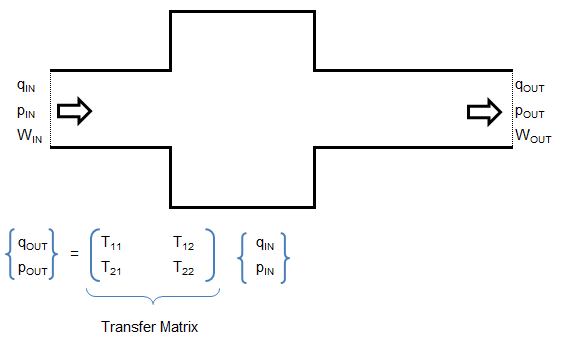Sometimes acoustic mass velocity is used instead of acoustic 'volume' velocity where mass velocity = ρ × volume velocity.
• Step-3: Overall TL of the system is product of TL of each sub-system in series.

TMM for a duct: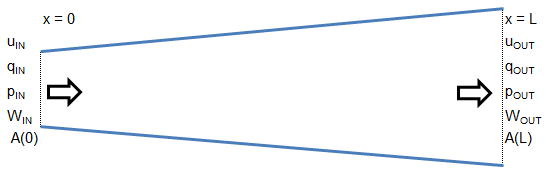The equation of a (plane) wave at any axial location is superposition of waves traveling along +x and -x direction and given by: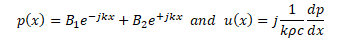Note that we have 4 unknowns assuming that the acoustic pressure and velocity at one the ends of the duct is known. Using continuity (mass conservation) and solving for B1 and B2 in terms of p(0) and u(0) then substituting into equations for p(L) and u(L):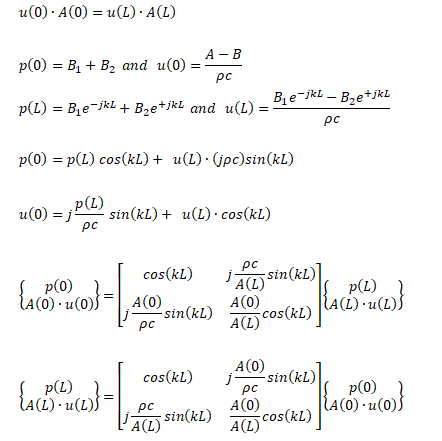Similarly, duct with absorptive material having complex wave number kc and complex characteristic impedance Zc:Sudden expansion and contraction: from continuity and equality of pressure on the two sides of the expansion or contraction plane, p(0) = p(L) and u(0)A(0)=u(L)A(L). Thus, transfer matrix is given by: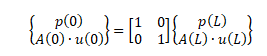Since, an expansion chamber = sudden expansion + duct + sudden contraction, the transfer matrix for an expansion chamber 'excluding' inlet and outlet ducts is given by: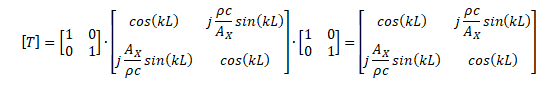#### Noise Level Regulations

NR: The permissible exposure limit (PEL) is a combination of sound pressure level and time of exposure. As per OSHA Hearing Conservation Amendment (HCA) 1981–1983, sound pressure level of 80 dBA has time limit of 32 hours whereas a level of 110 dBA has time limit of only 0.5 hours.
• Determination of sound power levels of noise sources — Guidelines for the use of basic standards: ISO 1680:2013
• Test code for the measurement of airborne noise emitted by rotating electrical machines: ISO 1680:2013
• Measurement of sound pressure level from service equipment in buildings — Engineering method: ISO 16032:2004
• Requirements for the performance and calibration of reference sound sources used for the determination of sound power levels: ISO 6926:2016
• Determination of sound power levels and sound energy levels of noise sources using sound pressure — Precision methods for reverberation test rooms: ISO 3741:2010
• Determination of sound power levels and sound energy levels of noise sources using sound pressure — Engineering methods for an essentially free field over a reflecting plane: ISO 3744:2010
• Determination of sound power levels and sound energy levels of noise sources using sound pressure — Precision methods for anechoic rooms and hemi-anechoic rooms: ISO 3745:2012
• Attenuation of sound during propagation outdoors — Part 1: Calculation of the absorption of sound by the atmosphere: ISO 9613-1:1993
• Determination of sound power levels of noise sources using sound intensity — Part 3: Precision method for measurement by scanning: ISO 9614-3
• Pass-by Noise: ISO 7216:1992
• Noise for agriculture and forestry wheeled tractors in motion fitted with elastic tyres or rubber tracks: governed by standard ISO 7216:2015. Test method specified by this standard require an acoustical environment which can only be obtained in an wide open space. Noise limits:
• Tractor with no load condition < 1.5 ton: 85 dB
• Tractor with no load condition ≥ 1.5 ton: 89 dB
• Construction equipments
• Noise level at operator's position at stationary condition is governed by ISO 6394:2008. For exterior locations, ISO 6393:2008 applies.
• Noise level at operator's position under dynamic condition is governed by ISO 6396:2008. For exterior locations, ISO 6395:2008 applies.
##### NC Curve
Noise Criteria (NC) rates the indoor background noise levels in buildings and rooms. It is used to judge the appropriateness of the acoustic environment for various activities. The American Society of Heating, Refrigeration and Air Conditioning Engineers (ASHRAE) makes recommendations for the NC levels for various spaces such as Concert Halls, Conference room, Classrooms ...
##### Speech Interference Level (SIL)
Excessive background noise interferes with speech which causes annoyance and irritation. When verbal communication is disrupted, worker's efficiency can suffer and the likelihood of error due to miscommunication is increased. A-weighted sound level is the simplest method used to predict speech intelligibility. It is a plot to specify talked-to-listener distance for just-reliable communications. It is common for a person to raise his voice when the background noise is high. Typically, a person raises the voice by 3 ~ 6 [dB] for every 10 [dB] increase in background noise level above 50 dBA.
Frequency bands for octave and 1/3-octavesB- and -C-Wighting Functions: While dBA is used to emphasize the fact that low frequency and high frequency noise pose less risk to humans, dBC is used for selection of hearing protection devices.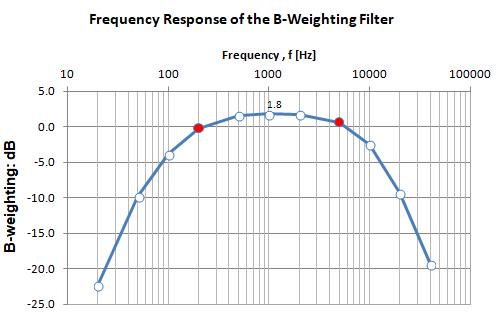The frequency weighting correction value in tabulate form is as follows:

 Centre Frequency Correction [dB] Frequency [hz] A-weighting C-weighting D-weighting 10 -70.4 -14.3 - 12.5 -63.4 -11.2 - 16 -56.7 -8.5 - 20 -50.5 -6.2 - 25 -44.7 -4.4 - 31.5 -39.4 -3.0 - 40 -34.6 -2.0 - 50 -30.2 -1.3 -12.8 63 -26.2 -0.8 -10.9 80 -22.5 -0.5 -9.0 100 -19.1 -0.3 -7.2 125 -16.1 -0.2 -5.5 160 -13.4 -0.1 -4.0 200 -10.9 0.0 -2.6 250 -8.6 0.0 -1.6 315 -6.6 0.0 -0.8 400 -4.8 0.0 -0.4 500 -3.2 0.0 -0.3 630 -1.9 0.0 -0.5 800 -0.8 0.0 -0.6 1000 0.0 0.0 0.0 1250 0.6 0.0 2.0 1600 1.0 -0.1 4.9 2000 1.2 -0.2 7.9 2500 1.3 -0.3 10.6 3150 1.2 -0.5 11.5 4000 1.0 -0.8 11.1 5000 0.5 -1.3 9.6 6300 -0.1 -2.0 7.6 8000 -1.1 -3.0 5.5 10000 -2.5 -4.4 3.4 12500 -4.3 -6.2 -1.4 16000 -6.6 -8.5 - 20000 -9.3 -11.2 -

##### Lighthill's Equation
Most of the derivations available in textbooks and literature on acoustics use Einstein's short-hand notation. Hence, a clear understanding of the convention is a strong prerequisite to understand each steps described below. The shorthand notation is based on concepts of "free index" and "dummy index".
• A free index appears once and only once within each additive term. The same letter must be used for the free index in every additive term.
• The first free index in a term corresponds to the row, and the second corresponds to the column. Thus, a vector (which has only one free index) is written as a column of three rows and a second order tensor is represented as 3 × 3 size matrix.
• A dummy index defines an index which does not appear in the final expression any more and implies a summation over the range of the index.
• A dummy index appears twice and exactly twice within an additive term of an expression.
• Number of free-index on left hand and right hand sides of an equation should be equal.

The continuity or mass conservation equation is given by:Navier-Stokes equation in vector form is expressed as:The same equation in scalar or x-, y- and z-component are written as set of 3 equations:

X-Momentum equation: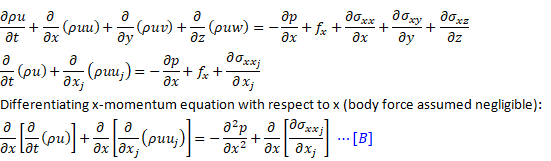Y-Momentum equation:Z-Momentum equation: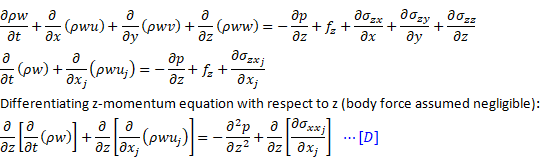In order to get a single scalar equation, the momentum equation also needs to be converted into a scalar equation. This is achieved by taking divergence of equation [ii]. Thus:Introducing pressure and density fluctuations:If the right hand side of Lighthill's equation is assumed to be known or estimated independent of the left hand side, this equation can be viewed as an inhomogeneous wave equation in an isotropic medium at rest. This also means that the sound propagation phenomena is not affected by sound generation. In flows with low Mach number, this assumption is useful and holds true and most aeroacoustic simulations are based on this simplification. Note that Lighthill's analogy does not account for presence of boundaries. This analogy is also classified as "density-based analogy" as the primary variable of the governing equation is the density of the medium.

Other variants of this equations have evolved such as Ffowcs-Williams and Hawkings equation that includes surfaces in motion. Thus, FWH analogy is used in solid surface interactions that are directly involved in the generation of flow sound, for example those due to rotors of a helicopter, propellers driving aeroplanes and marine vessels and prime movers like fans, compressors and turbines.

Similarly, a special version of FWH analogy is the Curle’s analogy that takes into account presence of solid rigid boundaries where only the dipole source terms takes care of the sound scattering caused by the stationary surface.

In addition to analogies presented above, numerous other analogies have been developed: Phillips’ analogy and Lilley’s analogy are based on scaled logarithmic pressure Π = 1/γ·ln[p'/p0]. These are also known as phi-based analogy and have been used as a starting point for aero-acoustics calculations of jet engines.

##### Acoustic perturbation equations: APE
Acoustic perturbation equations based on flow decomposition via source filtering by Ewert, R. ; Schröder, W. - Abstract: "A family of acoustic perturbation equations is derived for the simulation of flow-induced acoustic fields in time and space. The mean flow convection and refraction effects are part of the simulation of wave propagation. Using linearized acoustic perturbation equations the unbounded growth of hydrodynamic instabilities in critical mean flows is prevented completely. The perturbation equations are excited by source terms determined from a simulation of the compressible or the incompressible flow problem. Since the simulation of wave propagation contains the convection effects the computational domain of the flow simulation has to comprise only the significant acoustic source region. The acoustic perturbation equations are validated by computing a monopole source in a sheared mean flow, the sound generated due to a spinning vortex pair, and the sound generated by a cylinder in a crossflow."

Like any other acoustic analogy, here also the different scales of hydrodynamics and acoustics are handled by spliting the flow field into incompressible mean flow field and compressible acoustic part, also known as HAS (Hydrodynamics Acoustics Splitting) method. Thus: p = pIC + pAC, u = uIC + uAC and ρ = ρ0 + ρAC where speed of sound 'c' is defined as ρAC = c02pAC.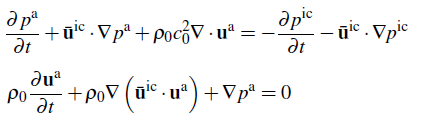##### Curle Analogy
Reference: "Implementation of Aeroacoustic Methods in OpenFOAM" by Erika Sjöberg. We have Lighthill's wave equation expressed as: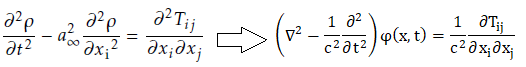Here a ≡ c. The acoustic wave equation can be solved analytically if the right hand side is assumed known and a common approach is to integrate the sources using a free fields Green function. Here, &tehta; is the retarded time i.e. the time at which the source radiates a fluctuation that reaches the observer at time 't', x is observation coordinates and y represents source coordinates and ρ0 is a constant of integration.Explanation on Green's function

An equation of the form L1ρ = L2q where L1 and L2 are linear differential operators, has a solution of the following form. Note that the L1 operator is the D'Alembert operator 𗡌2 is given by: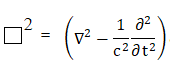ρ(x, t) = L2(qG) = L2q ∫q(y, θ) G(x - y, t-θ)dydθ where θ is the retarded time and y represents source coordinates. The Green's function G is defined as L1G = δ. The Green's function for the isotropic wave operator (L1) or D'Alembert operator 𗡌2 is: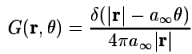The general solution of the inhomogeneous Lighthill's wave equation on a bounded domain is given below where n is the surface normal pointing towards the fluid.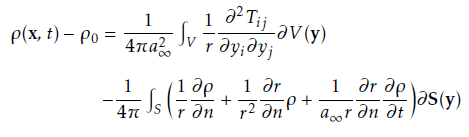Utilizing partial integration and symmetrical properties from the Green’s function G:The last term integral in previoud characterizes the monopole field created by fluid vibrations of the body which in many cases can be neglected. In the case of solid surfaces where the velocity on the surface is zero.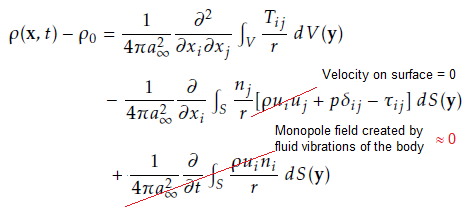Curle’s final equation simplifies to: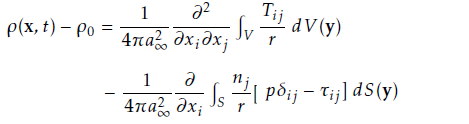Flow chart of OpenFOAM-Acoustic-Solver acousticFoam for incompressible simulations: reference - Implementation of Acoustic Analogies in OpenFOAM for Computation of Sound Fields by J. Schmalz, W. Kowalczyk, Chair of Mechanics and Robotics, University of Duisburg-Essen, Germany##### References
1. A Textbook of Sound: A. B. Wood
2. https://www.ncbi.nlm.nih.gov/pmc/articles/PMC4906304/
3. COMSOL: Acoustic Module User Guide
4. ANSYS FLUENT User Manual and Theory Guide
5. Computational Aeroacoustics Methods with OpenFOAM v. 4.1: V. Korchagova, M. Kraposhin, S. Strizhak
6. Open-Rotor Noise Assessment with CFD/CAA Chaining: A. Chelius, T. Le Garrec, D.C. Mincu, Onera - The French Aerospace Lab, F-92322, Chatillon, France
7. Foundations of acoustic analogies: Seppo Uosukainen, VTT Publications 757
8. "A-Weighting": Is it the metric you think it is?: McMinn, Terrance - Curtin University of Technology